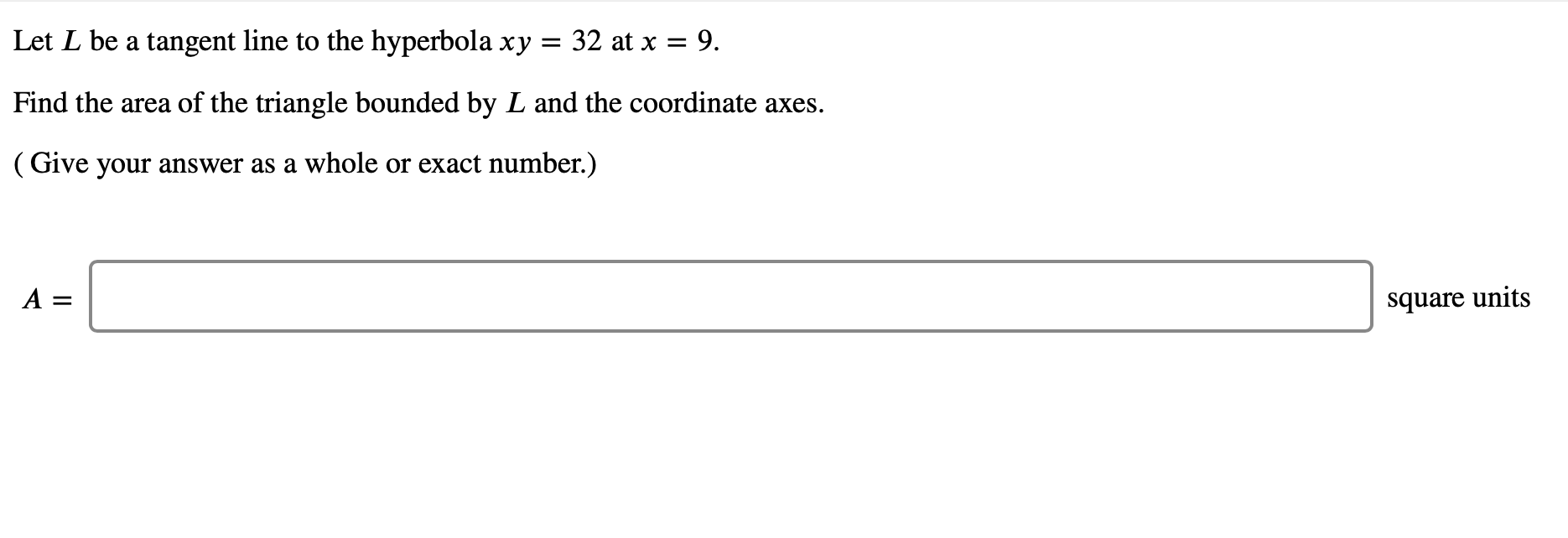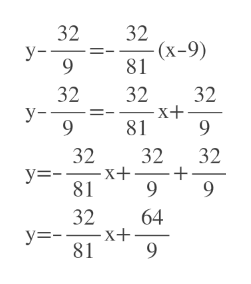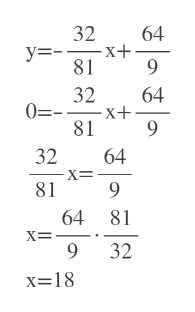# 32 at x 9Let L be a tangent line to the hyperbola xyFind the area of the triangle bounded by L and the coordinate axes(Give your answer as a whole or exact number.)A =square units

Questionhelp_outlineImage Transcriptionclose32 at x 9 Let L be a tangent line to the hyperbola xy Find the area of the triangle bounded by L and the coordinate axes (Give your answer as a whole or exact number.) A = square units fullscreen
check_circle

Step 1

We have to find slope at x=9

We need y'(9)

y'(9)= -32/81

Step 2

At x=9, y=32/9

So we know slope= -32/81 and point is (9,32/9)

We will use the point slope formula to find the tangent

Tangent is y= (-32/81)x+(64/9)help_outlineImage Transcriptionclose32 -(x-9) 81 32 у- 9 32 32 X+ 81 32 у- 9 9 32 X+ 81 32 32 у-- 9 9 32 64 -X+ 81 9 fullscreen
Step 3

y intercept of the tangent is 64/9

To find x intercept w...help_outlineImage Transcriptionclose32 64 y= x+ -X+ 81 9 64 32 81 32 64 X= 9 81 64 81 32 9 x 18 fullscreen

### Want to see the full answer?

See Solution

#### Want to see this answer and more?

Solutions are written by subject experts who are available 24/7. Questions are typically answered within 1 hour.*

See Solution
*Response times may vary by subject and question.
Tagged in

### Other